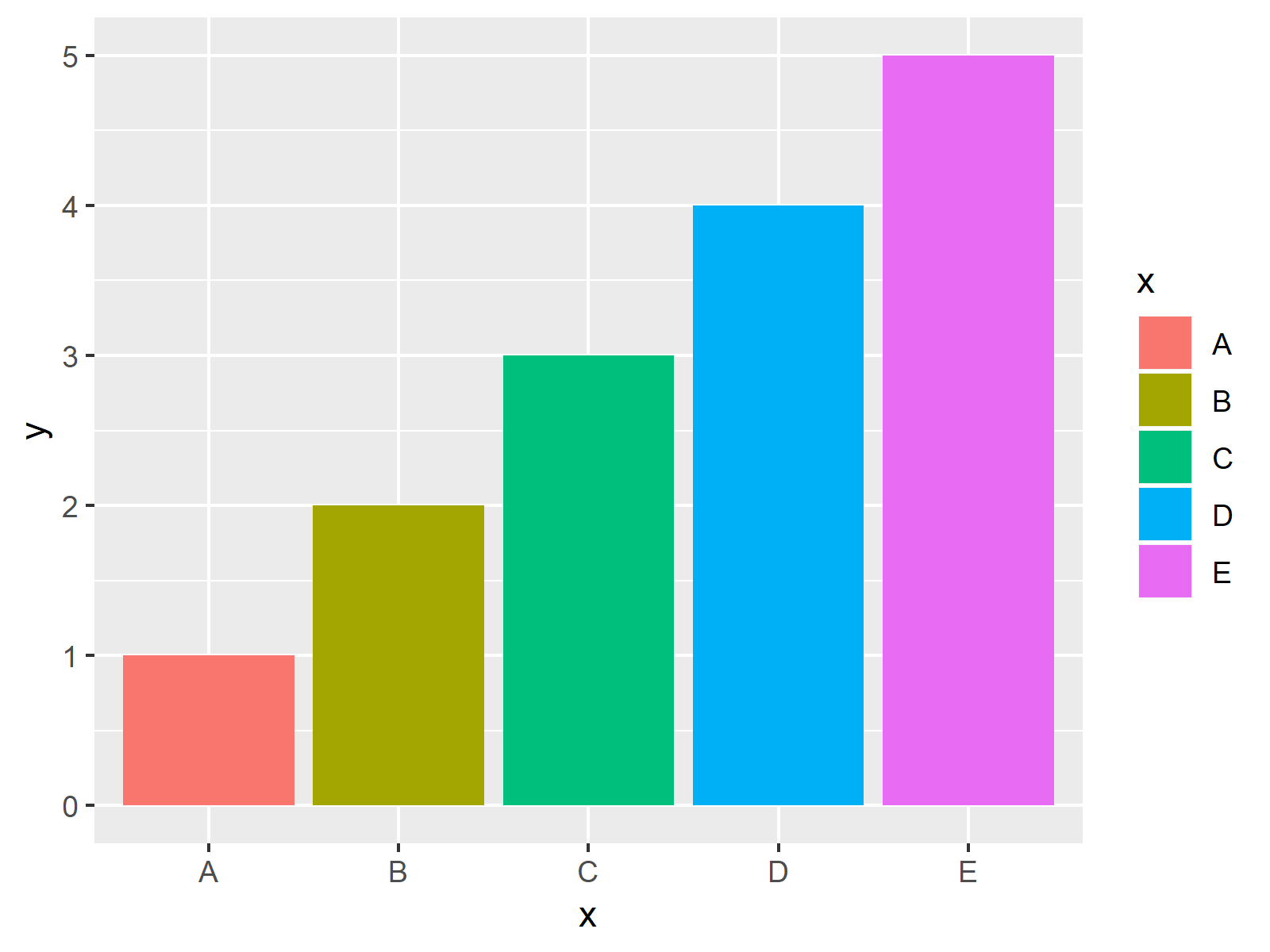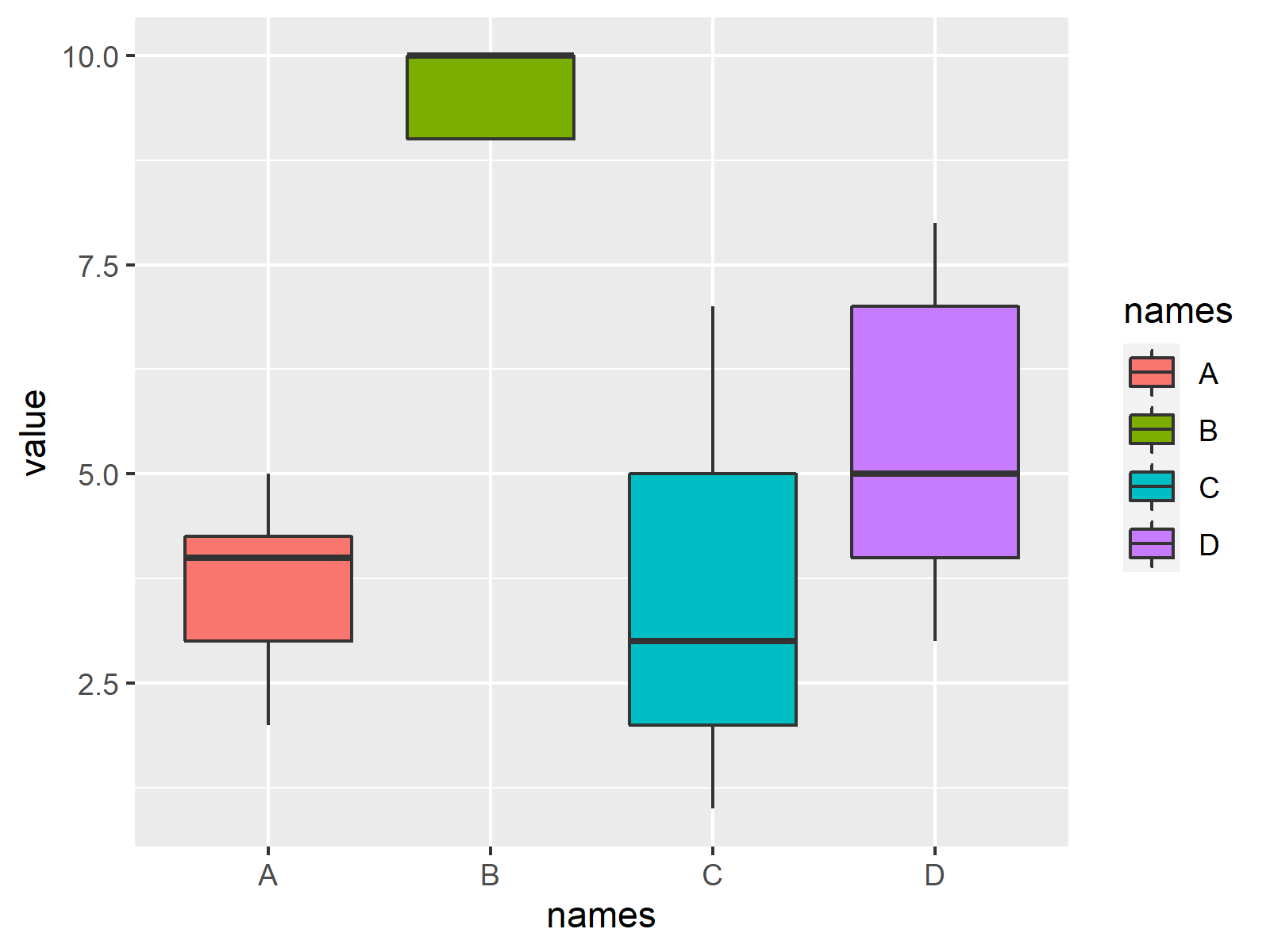# R ggplot2 Error: Aesthetics must be either length 1 or the same as the data

In this tutorial you’ll learn how to fix the error message “Aesthetics must be either length 1 or the same as the data” in the R programming language.

Let’s start right away…

## Example Data, Add-On Packages & Default Graph

As a first step, we’ll need to create some data that we can use in the examples later on:

```data <- data.frame(x = LETTERS[1:5],    # Create example data
y = 1:5)
data                                    # Print example data
#   x y
# 1 A 1
# 2 B 2
# 3 C 3
# 4 D 4
# 5 E 5```

In case we want to draw our data with the ggplot2 package, we also have to install and load ggplot2 to R:

```install.packages("ggplot2")             # Install & load ggplot2 package
library("ggplot2")```

## Example 1: Reproducing the Error: Aesthetics must be either length 1 or the same as the data

In Example 1, I’ll show how to replicate the error message “Aesthetics must be either length 1 or the same as the data” in R.

Have a look at the following R code:

```ggplot(data, aes(x, y, fill = c("red", "blue"))) + # Try to draw ggplot2 plot
geom_bar(stat = "identity")
# Error: Aesthetics must be either length 1 or the same as the data (5): fill```

As you can see, the RStudio console returns the error “”Aesthetics must be either length 1 or the same as the data”.

The reason for this is that we have specified the fill argument within the aes function to be equal a vector of length 2 (i.e. c(“red”, “blue”)).

However, our example data has five categories and therefore doesn’t know which filling color should be used for which category.

Let’s solve this problem!

## Example 2: Fixing the Error: Aesthetics must be either length 1 or the same as the data

This example shows how to deal with the ggplot2 error “Aesthetics must be either length 1 or the same as the data”.

As discussed before, we have to specify a filling color for each group in our data. The easiest way to do this is that we set fill to be equal to our grouping variable (i.e. x):

```ggplot(data, aes(x, y, fill = x)) +     # Properly drawing ggplot2 plot
geom_bar(stat = "identity")```As illustrated in Figure 1, the previous syntax created a ggplot2 barplot in R. Looks good!

## Video & Further Resources

I have recently released a video on my YouTube channel, which shows the R programming syntax of this tutorial. Please find the video below:

Please accept YouTube cookies to play this video. By accepting you will be accessing content from YouTube, a service provided by an external third party.If you accept this notice, your choice will be saved and the page will refresh.

Furthermore, I can recommend to have a look at the other articles of my homepage.

Summary: You learned in this article how to handle the error “Aesthetics must be either length 1 or the same as the data” in R programming. In case you have any further questions, let me know in the comments.

Subscribe to the Statistics Globe Newsletter

•Ananya Sharma
January 10, 2022 5:14 pm

Hi,
I was trying to do the following code, and it hasn’t worked out.
I have picked the inbuilt set mpg and also run the suitable packages.
I get the error: Error: Aesthetics must be either length 1 or the same as the data (234): x, y and fill

set.seed(10)
names<-c(rep("A",20),
rep("B",5),
rep("C",30),
rep("D",100))
value<- c(sample(2:5, 20, replace=T),
sample(4:10, 5, replace=T),
sample(1:7, 30, replace=T),
sample(3:8, 100 ,replace=T))

data1<-data.frame(names,value)
data1
ggplot(data=mpg,(aes(x=names,y=value,fill=names))) +
geom_boxplot( stat="identity")

Thanks and kind regards,
Ananya

•January 10, 2022 5:30 pm

Hey Ananya,

Your code works for me when I do the following changes:

• Replace mpg by data1
• Delete stat=”identity”

My final code looks like this:

```ggplot(data=data1,(aes(x=names,y=value,fill=names))) +
geom_boxplot()```

And the resulting plot looks like this:Regards,
Joachim

•Charlotte
March 9, 2022 4:06 am

CHD <- rawdata\$anychd
CHD2 <- as.character(CHD)
prev_CHD <- factor(CHD2, levels = c(0,1), labels = c("NO", "YES"))
age <- rawdata\$AGE

ggplot(rawdata, aes(x=prev_CHD, y=age, coll = prev_CHD)) + geom_boxplot() + theme(legend.position = "none") + xlab("CHD Event") +ylab("Age (years)") + ggtitle("Relationship Between Age & Presence of CHD Event")

I also get the same error and was wondeirng why? Thanks!

•March 10, 2022 8:23 am

Hey Charlotte,

You should store all the relevant information for the plot within a single data frame, not in separate data objects.

You may create a data frame containing prev_CHD and age and run the code again based on this data frame.

Regards,
Joachim

•Mike P
June 1, 2022 11:25 pm

Hello,
I am just trying to generate a GSEA plot via plotEnrichment(). I did the following:

plotEnrichment(examplePathways[[“5991130_Programmed_Cell_Death”]],
exampleRanks) + labs(title=”Programmed Cell Death”)

Error in `check_aesthetics()`:
! Aesthetics must be either length 1 or the same as the data (1): x and xend
Run `rlang::last_error()` to see where the error occurred.

•June 6, 2022 4:37 pm

Hey Mike,

I’m sorry for the delayed response, I’ve been on vacation for the last couple of days. Do you still need help with this error message?

Regards,
Joachim

•Milana
September 8, 2022 3:07 am

Hi, I am trying to produce a forest plot for my regression analysis using the following code:

plot1 = ggplot(output6_0b[] %>%
mutate(ci.lb = ifelse(ci.lb cutoff_upper,
cutoff_upper, ci.ub)) ) +
geom_point(aes(y = name, x = estimate),
shape = 18, size = 5) +
geom_errorbarh(aes(xmin = ci.lb, xmax = ci.ub,
y = name), height = 0.25) +
geom_vline(xintercept = 1, color = “red”,
linetype = “dashed”, cex = 1, alpha = 0.5) +
# scale_x_continuous(limit = c(1.01*cutoff_lower, 1.01*cutoff_upper) ) +
xlab(“Lnrr”) +
ylab(“”) +
theme_bw() +
theme(panel.border = element_blank(),
panel.background = element_blank(),
panel.grid.major = element_blank(),
panel.grid.minor = element_blank(),
axis.line = element_line(colour = “black”),
axis.text.y = element_text(size = 12, colour = “black”),
axis.text.x.bottom = element_text(size = 12, colour = “black”),
axis.title.x = element_text(size = 12, colour = “black”))

table_base <- ggplot(output6_0b[], aes(y=name)) +
ylab(NULL) + xlab(" ") +
theme(plot.title = element_text(hjust = 0.5, size=12),
axis.text.x = element_text(color="white", hjust = -3, size = 25), ## This is used to help with alignment
axis.line = element_blank(),
axis.text.y = element_blank(),
axis.ticks = element_blank(),
axis.title.y = element_blank(),
legend.position = "none",
panel.background = element_blank(),
panel.border = element_blank(),
panel.grid.major = element_blank(),
panel.grid.minor = element_blank(),
plot.background = element_blank())

## OR point estimate table
tab1 <- table_base +
labs(title = "space") +
geom_text(aes(y = rev(name), x = 1, label = sprintf("%0.1f", round(estimate, digits = 1))), size = 4) + ## decimal places
ggtitle("lnrr")

## 95% CI table
tab2 <- table_base +
geom_text(aes(y = rev(name), x = 4, label = CI), size = 4) +
ggtitle("95% CI")

lay <- matrix(c(1,1,1,1,1,1,1,1,1,1,2,3,3), nrow = 1)
grid.arrange(plot1, tab1, tab2, layout_matrix = lay)

but when I run the last line of the code I get the same error. I tried to find out the error but unfortunately could not. Could you please suggest why this error might appear and how to deal with it in this case? Thank you

•September 8, 2022 6:46 am

Hi Milana,

This is difficult to tell without seeing your data. I assume output6_0b[] is a data.frame object? Could you please share the output when you run the following code?

```head(output6_0b[])
str(output6_0b[])```

Regards,
Joachim

•Milana
September 8, 2022 11:04 am

Hi Joachim,

Here they are

# A tibble: 4 × 7
name estimate se zval pval ci.lb ci.ub

1 intrcpt 2.98 2.10 1.42 0.156 -1.13 7.09
2 duration_exp -0.0387 0.0226 -1.71 0.0868 -0.0830 0.00559
3 application_rate -0.00554 0.0140 -0.395 0.693 -0.0330 0.0220
4 fertilizer_app_rate 0.000688 0.000911 0.755 0.450 -0.00110 0.00247

> str(output6_0b[])
tibble [4 × 7] (S3: tbl_df/tbl/data.frame)
\$ name : chr [1:4] “intrcpt” “duration_exp” “application_rate” “fertilizer_app_rate”
\$ estimate: num [1:4] 2.980461 -0.038696 -0.005538 0.000688
\$ se : num [1:4] 2.098382 0.022598 0.014032 0.000911
\$ zval : num [1:4] 1.42 -1.712 -0.395 0.755
\$ pval : num [1:4] 0.1555 0.0868 0.6931 0.4502
\$ ci.lb : num [1:4] -1.1323 -0.083 -0.033 -0.0011
\$ ci.ub : num [1:4] 7.09321 0.00559 0.02196 0.00247

Regards,
Milana

•September 9, 2022 6:44 am

Hey Milana,

Thank you for the additional info.

I have tried to reproduce your data as shown below:

```library("dplyr")

set.seed(367854)
output6_0b <- list()
output6_0b[] <- tibble(data.frame(name = sample(letters[1:4], replace = TRUE),
estimate = rnorm(100),
se = rnorm(100),
zval = rnorm(100),
pval = rnorm(100),
ci.lb = rnorm(100),
ci.ub = rnorm(100)))
# # A tibble: 6 x 7
#  name  estimate     se   zval    pval  ci.lb  ci.ub
#   <chr>    <dbl>  <dbl>  <dbl>   <dbl>  <dbl>  <dbl>
# 1 b       -0.298  1.40   0.748  1.54    0.854 -0.552
# 2 b       -0.877  1.84   0.549 -1.15    0.712 -0.246
# 3 b       -1.88   1.50  -1.07   0.0486  0.863 -1.68
# 4 d       -0.593 -0.786 -0.523 -0.289   1.97  -1.45
# 5 b       -0.162  0.234  1.68  -0.184   0.171  0.693
# 6 b       -1.05   1.35  -0.229  0.134  -1.03  -1.23```

However, when I now run the first lines of your code, I get an error due to the data object cutoff_upper. Where is this data object coming from?

Furthermore, it seems like there is something wrong with the specification of the ifelse function: ifelse(ci.lb cutoff_upper, cutoff_upper, ci.ub) Why is there a space between ci.lb & cutoff_upper?

Regards,
Joachim

•alii
February 18, 2023 7:36 pm

Hi, could you please check where I made error. I am trying to created graph with forecast.

My code:
ggplot(data=myxts_final,aes (x=index(myxts_final)))+
ggtitle(“FTSE Close Index Forecasts”)+
geom_ribbon(aes(ymin = y.lo80, ymax = y.hi80),fill = “blue”, alpha = 0.2)+
geom_ribbon(aes(ymin = y.lo95, ymax = y.hi95),fill = “blue”, alpha = 0.2)+
theme(axis.title.x=element_blank(),
axis.title.y=element_blank(),
axis.text.x=element_text(color=”black”,size=12),
axis.text.y=element_text(color=”black”, size=12),
panel.background=element_rect(fill=”white”),
panel.grid.minor=element_blank(),
panel.grid.major=element_blank(),
axis.line=element_line(color=”black”, size=1),
plot.title=element_text(face=”bold”))+ggeasy::easy_center_title()

The error I get:
Don’t know how to automatically pick scale for object of type .
Defaulting to continuous.
Error in `geom_line()`:
! Problem while computing aesthetics.
ℹ Error occurred in the 1st layer.
Caused by error in `check_aesthetics()`:
! Aesthetics must be either length 1 or the same as the data (6613)
✖ Fix the following mappings: `y`

Thank you!

•February 21, 2023 9:47 am